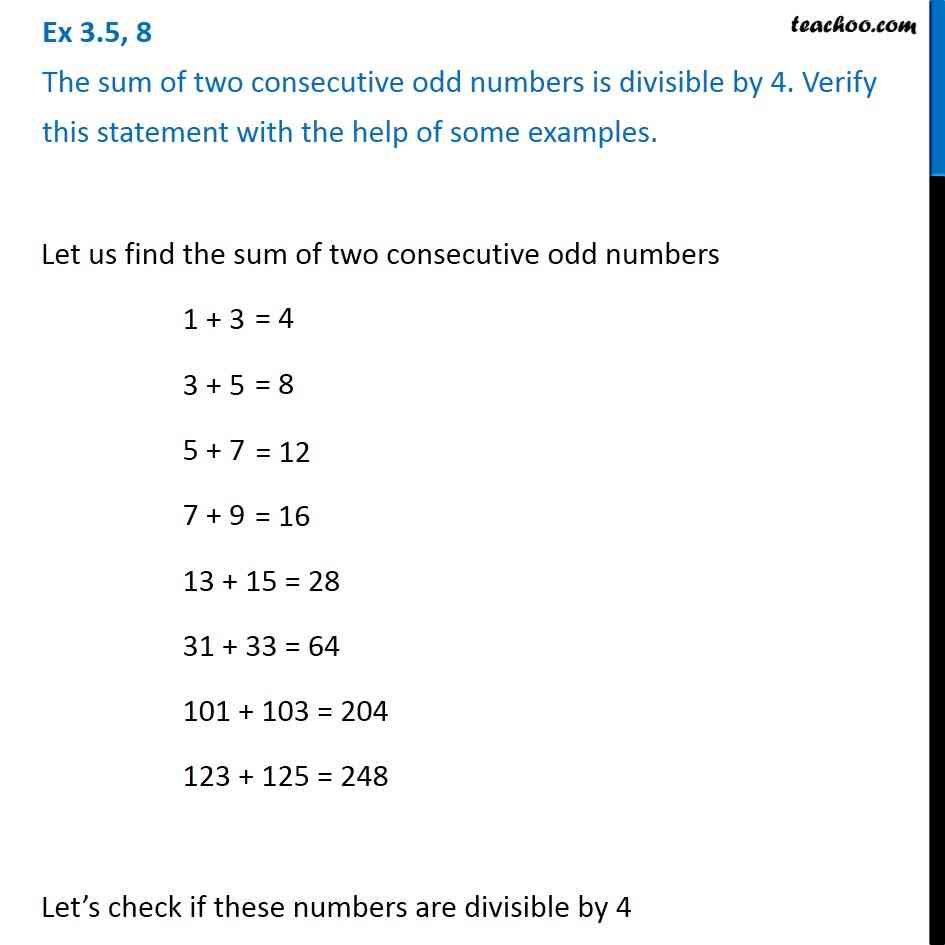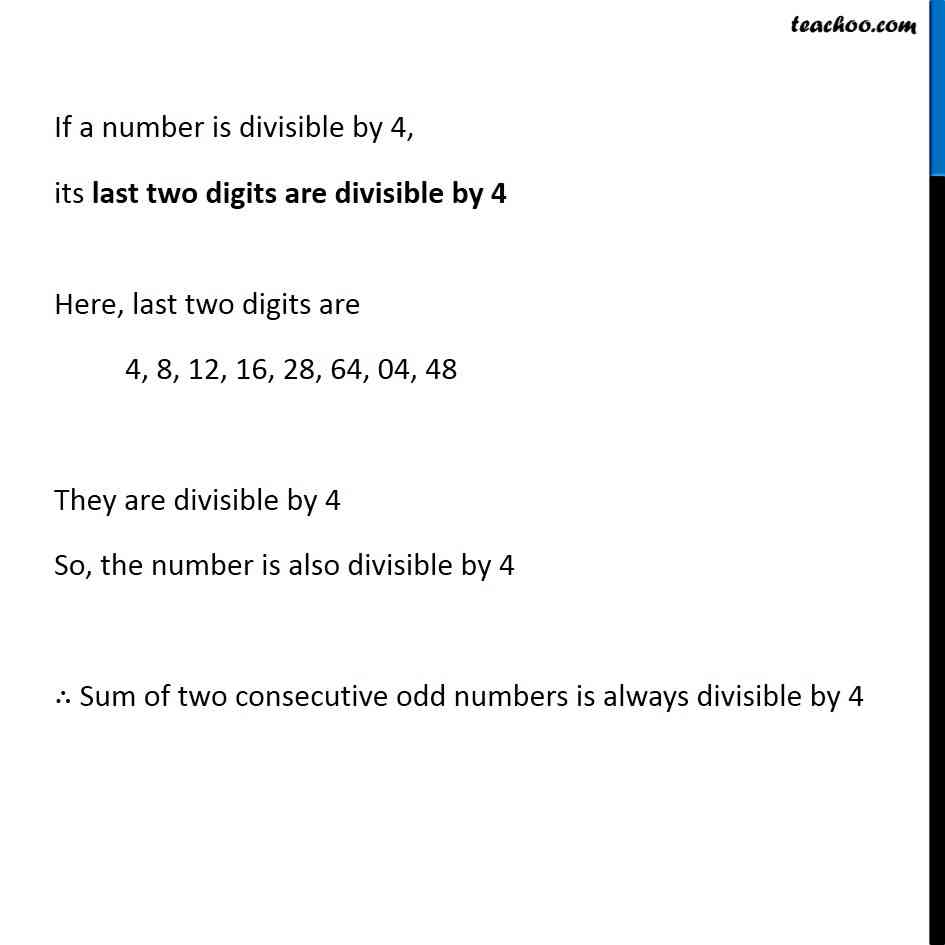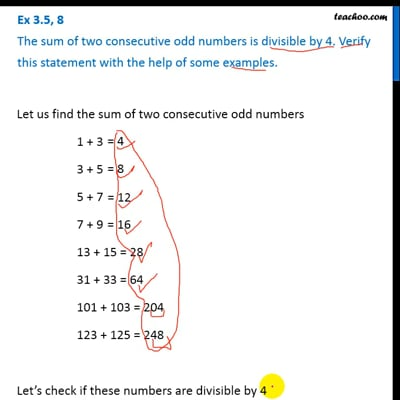More Divisibility Rules

Chapter 3 Class 6 Playing with Numbers
Concept wiseThis video is only available for Teachoo black users

Introducing your new favourite teacher - Teachoo Black, at only ₹83 per month

### Transcript

Ex 3.5, 8 The sum of two consecutive odd numbers is divisible by 4. Verify this statement with the help of some examples.Let us find the sum of two consecutive odd numbers 1 + 3 3 + 5 5 + 7 7 + 9 13 + 15 = 28 31 + 33 = 64 101 + 103 = 204 123 + 125 = 248 Let’s check if these numbers are divisible by 4 If a number is divisible by 4, its last two digits are divisible by 4 Here, last two digits are 4, 8, 12, 16, 28, 64, 04, 48 They are divisible by 4 So, the number is also divisible by 4 ∴ Sum of two consecutive odd numbers is always divisible by 4